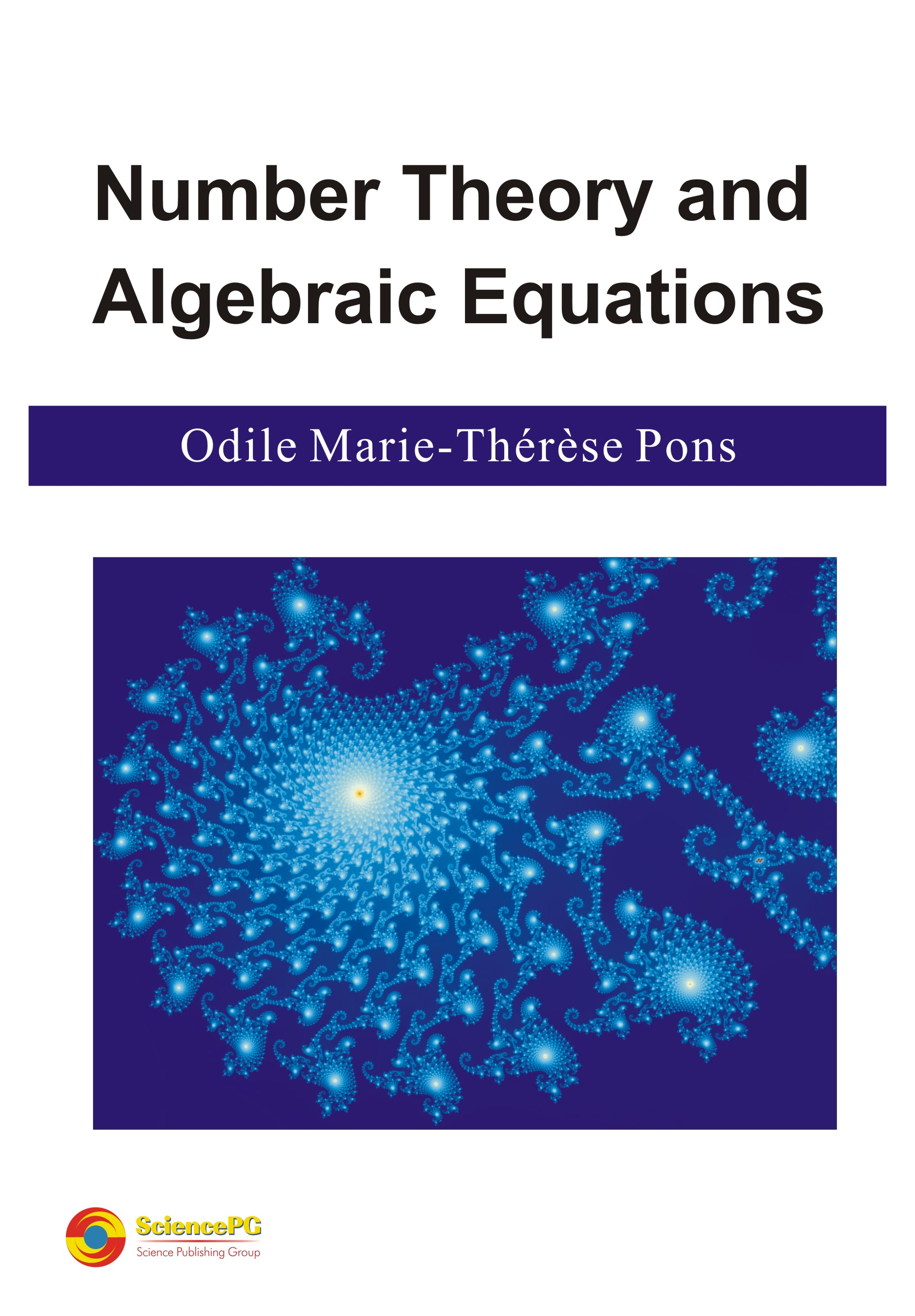Closed ×
Home / Books / Published Books / Number Theory and Algebraic EquationsNumber Theory and Algebraic Equations
Author:
Odile Marie-Thérèse Pons
ISBN:
978-1-940366-74-6
Published Date:
November, 2016
Pages:
240
Paperback:
\$120
E-book:
\$25
Publisher:
Science Publishing GroupSEMI-OPEN ACCESSThe Whole Book
Front Matter
Since November 3, 2016
Chapter 1 Introduction
Since November 3, 2016
1.1 Factorization of the Integers
1.2 Polygonal Numbers
1.5 Exercises
Chapter 2 Fermat’s First Theorem and Quadratic Residues
Since November 3, 2016
2.1 Fermat’s First Theorem
2.2 Divisors of an Integer
2.4 Wilson’s Theorem and Sums of Squares
2.5 Euler’s φ(n)
2.6 Exercises
Chapter 3 Algebraic Equations and Fermat’s Last Theorem
Since November 3, 2016
3.1 Algebraic Equations
3.2 Fermat’s Last Theorem
3.3 Catalan’s Equation
3.4 Generalizations of Fermat’s Last Theorem
3.5 Exercises
Chapter 4 Prime Numbers and Irrational Numbers
Since November 3, 2016
4.1 Cardinal of the Primes in Intervals
4.4 Algebraic Numbers of Degree n
4.5 Equations with Several Variables
4.6 Twin-Primes
4.7 Exercises
Chapter 5 Euler’s Functions
5.1 Definition
5.2 Approximations of the Function π (x)
5.3 Approximations of the Function Gamma
5.4 Riemann’s Equations for ζs
5.5 The Alternating Series
5.6 Bernoulli Polynomials
5.7 Trigonomeric Expansions
5.8 The Hurwitz Zeta Function
5.9 Logarithm of ζs
5.10 Exercises
Chapter 6 Automorphisms
6.1 Rings
6.2 Algebraic Extension of a Field
6.3 Galois’s Extension
6.4 Galois’s Theory
6.6 Exercises
Chapter 7 Functional Equations
7.1 Fermat's First Theorem for Polynomials
7.2 Polynomials in Fp
7.4 Complex Algebraic Fields
7.5 Exercises
Chapter 8 Solutions of the Exercises
8.1 Introduction
8.2 Fermat’s First Theorem and Quadratic Residues
8.3 Algebraic Equations and Fermat’s Last Theorem
8.4 Prime Numbers and Irrational Numbers
8.5 The Function Zeta
8.6 Automorphisms
8.7 Functional Equations
Back Matter
Since November 3, 2016
Author(s)
Odile Marie-Thérèse Pons is a Senior Research Fellow in Mathematics at the French National Institute for Agronomical Research. She holds MSc., doctor’s degree and habilitation qualification in Mathematics at the university of Paris. She is the author of about a hundred of peer-reviewed articles in mathematical and applied statistics and probability, and 8 books in Probability, Statistics and Analysis.
Description
The book covers the classical number theory of the 17-19th centuries with simple algebraic proofs: theorems published by Fermat (his Last Theorem), Euler, Wilson, Diophantine equations, Lagrange and Legendre Theorems on the representation of integers as sums of squares and other classes of numbers, the factorization of polynomials, Catalan’s and Pell’s equations. It provides proofs of several conjectures and new generalizations of the classical theory, with applications to the prime numbers and polynomials.

A second part concerns the expansions in series of functions related to the cardinal of the primes, the functions Gamma and zeta. Several known formulas and new results are proved.

A third part introduces the algebra of rings and fields in relation to the theory the polynomials and Galois’s theory, which are applied to the resolution of algebraic equations. The classical theorems are extended to polynomials and to the complex field. Numerical examples and approximately 50 exercises with proofs illustrate the main topics.
PUBLICATION SERVICES